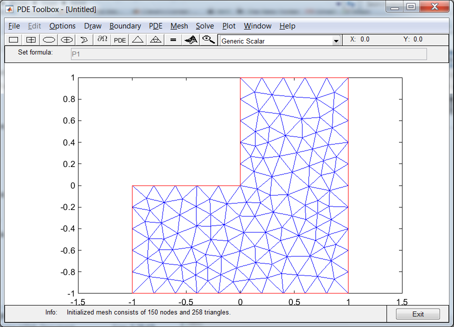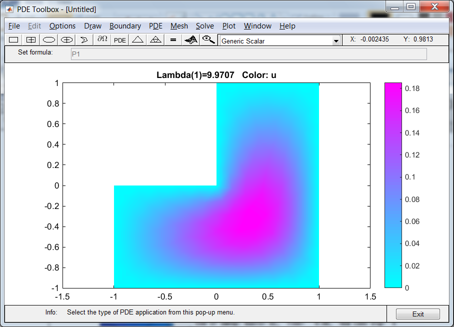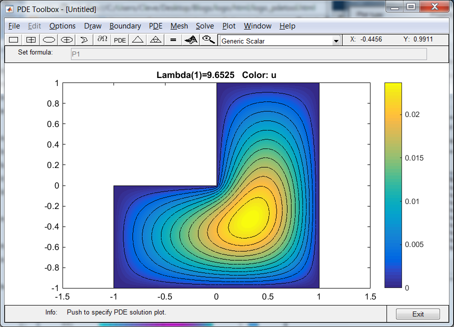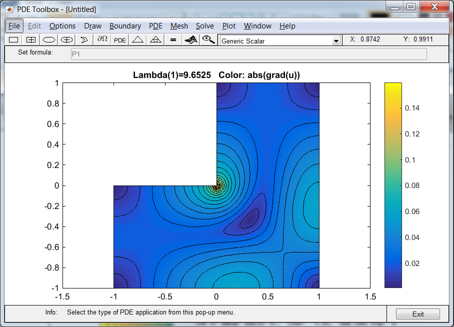# MathWorks Logo, Part Three, PDE Toolbox

The Partial Differential Equation Toolbox contains tools for the analysis of PDEs in two space dimensions and time. It is perhaps not surprising that one of the primary examples involves the L-shaped membrane.

### Contents

#### pdetool

If you have the PDE toolbox installed, bring up pdetool. Click on the first triangle in the toolbar. This initializes a tool for creating a planar domain with an unstructured mesh. The default domain is our L. The default mesh has equally spaced points on the boundary connected by an irregular grid in the interior with 150 nodes and 258 triangles.#### Coarse grid

The PDE tab allows the specification of a general elliptic operator with variable coefficients, but if we accept the defaults we have our desired Laplacian. Here is the first eigenfunction, obtained with the coarse grid, plotted with the default cool colormap. The first eigenvalue, reported in the title, is 9.9707.#### Finer grid

Refine the grid three times to one with 8417 nodes and 16512 triangles, change to our new parula colormap, and add contour lines. The reported eigenvalue is 9.6525.The pdetool has an option to plot the absolute value of the gradient. We see that the singularity at the origin acts like a black hole, sucking in all the color.#### Eigenvalues

The mesh refinement is accomplished by adding grid points at the midpoints of the triangles, thereby quadrupling the number of triangles. It is possible to do this five times, producing a fine mesh with 258 * 4^5 = 264192 triangles. (Actually the mesh can be refined six times to one with over a million triangles, but the subsequent eigenvalue calculation runs out of memory.)

Here are the results for the first eigenvalue of the L-shaped membrane obtained with successive bisections of the unstructured triangular mesh.

   format long
L

L =
9.970747465677787
9.745769128365856
9.675647712787020
9.652453140975609
9.644395207950412
9.641482807142362


#### Aitken delta-squared acceleration

These values are candidates for acceleration by Aitken's delta-squared process.

$$x_n - \frac {(\Delta x_n)^2}{\Delta^2 x_n}$$

   d1 = diff(L,1);
L2 = L(3:end) - d1(2:end).^2./diff(L,2)

L2 =
9.643895738979518
9.640988739828559
9.640105597452624
9.639834371546435


We can even use delta-squared a second time, although this is not often justified.

   t1 = diff(L2,1);
L2(3:end) - t1(2:end).^2./diff(L2,2)

ans =
9.639720224107141
9.639714153353552


Compare this with the value I reported in my previous post, obtained by extrapolating from a regular square grid.

lambda1 =
9.639723753239963


We will see in my next post a much better way to compute the eigenvalue that will provide a result to nearly full double precision accuracy.

Published with MATLAB® R2014b

|